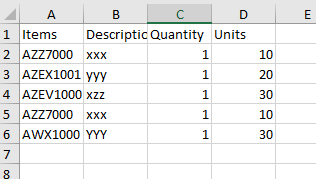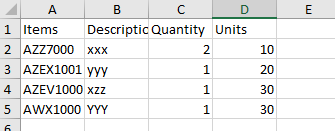# Need a Excel manipulation SolutionAttached is the Excel Data,

My task would be, if the Items column has the same values, to add the Quantity Values and remove the duplicate one from the excel

The expected output would be,@Iswarya_P1

Use this query

Datatablevaraible.AsEnumerable.GroupBy(Function(r) r(0).tostring).select(function(grp) grp.first).CopyToDataTable

r(0) is the index of the first columns

In general we can handle it as a Group by case

from the table we would derive (as AZZ700 is not summing up the Units) that the grouping is to be done over Items, Descr and Units Columns

Assign Activity
dtResult = dtOrig.Clone

Assign Activity
dtResult =

``````(From d in dtOrig.AsEnumerable
Group d by k1=d("Items").toString.Trim,k2=d("Descriptions").toString.Trim,k3=d("Units").toString.Trim into grp=Group
Let cnt = grp.Sum(Function (x) CInt(x("Quantity").toString.Trim))
Let ra = new Object(){k1, k2, cnt, k3}